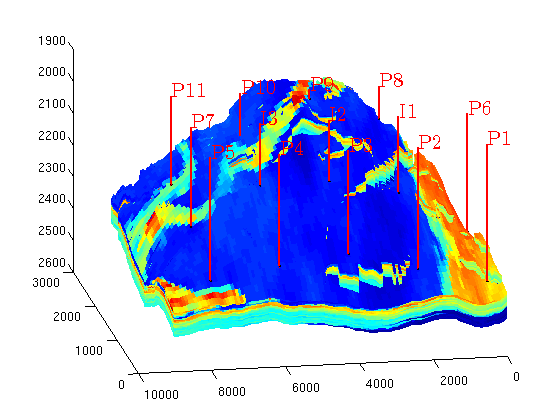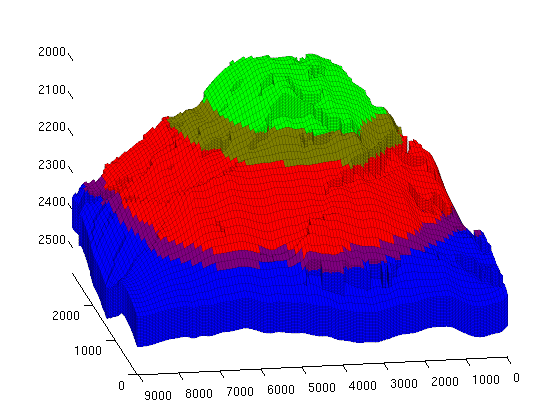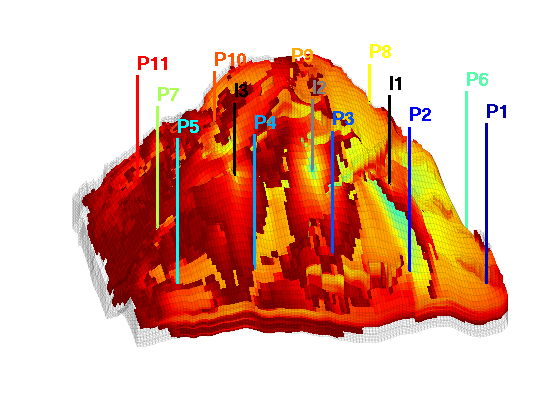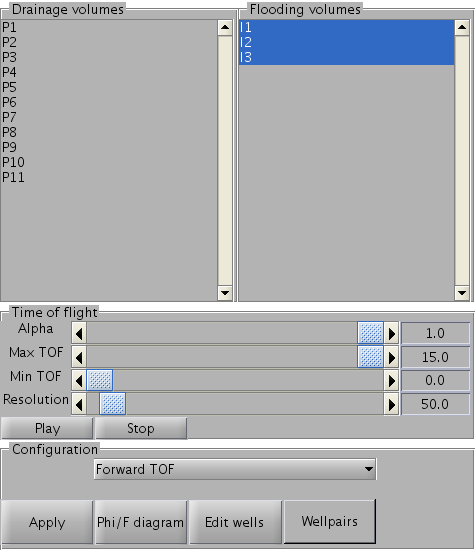#Interactive diagnostics on corner point grid
This example sets up interactive diagnostics on a corner point grid. The example uses a geological model of a shallow-marine reservoir generated by the SAIGUP modelling project and includes a number of arbitrary placed wells. The example is short, as the primary purpose is to create the interactive figures produced by interactiveDiagnostics.

## Set up grid and rock

```mrstModule add diagnostics gridtools mrst-gui
grdecl = fullfile(ROOTDIR, 'examples', 'data', 'SAIGUP', 'SAIGUP.GRDECL');

if ~exist(grdecl, 'file'),
error('SAIGUP model data is not available.')
end

actnum        = grdecl.ACTNUM;
grdecl.ACTNUM = ones(prod(grdecl.cartDims),1);
G             = processGRDECL(grdecl, 'checkgrid', false);
G             = computeGeometry(G(1));

rock = grdecl2Rock(grdecl, G.cells.indexMap);
is_pos                = rock.perm(:, 3) > 0;
rock.perm(~is_pos, 3) = min(rock.perm(is_pos, 3));
rock.perm = rock.perm.*milli*darcy;
```

## Set up wells

The wells are simply placed by a double for loop over the logical indices. The resulting wells give producers along the edges of the domain and injectors near the center.

```pv = poreVolume(G, rock);
ijk = gridLogicalIndices(G);
W = [];
gcz = G.cells.centroids;
[pi,ii] = deal(1);
for i = 5:12:G.cartDims(1)
for j = 5:25:G.cartDims(2)
c = ijk{1} == i & ijk{2} == j;
if any(c)
c = find(c);
x = gcz(c(1), 1); y = gcz(c(1), 2);
if x > 500 && x < 2000 && y < 7000 && y > 1000
% Set up rate controlled injectors for cells in the middle
% of the domain
val = sum(pv)/(1000*day);
type = 'rate';
name = ['I' num2str(ii)];
ii = ii + 1;
else
% Set up BHP controlled producers at the boundary of the
% domain
val = 250*barsa;
type = 'bhp';
name = ['P' num2str(pi)];
pi = pi + 1;
end

W = addWell(W, G, rock, c, 'Type', type, 'Val', val, 'Name', name);
end
end
end
% Plot the resulting well setup
clf
plotCellData(G, rock.poro),
plotWell(G, W)
view(-100, 25)
```## Set up a reservoir state

This is not strictly needed for the diagnostics application, but it allows us to see the reservoir component distribution around producers grouped by time of flight. The distribution of phases is done based on a simple hydrostatic approximation by using cell centroids. Some phase mixing is present.

```state = initResSol(G, 200*barsa, [0 0 0]);

gcz = G.cells.centroids(:, 3);
height = max(gcz) - min(gcz);
pos = 1 - (gcz - min(gcz))./height;

oil = pos > 0.4 & pos < 0.8;
gas = pos > 0.7;
wat = pos < 0.45;

state.s(wat, 1) = 1;
state.s(oil, 2) = 1;
state.s(gas, 3) = 1;

state.s = state.s ./ repmat(sum(state.s, 2), 1, 3);

clf;
rgb = @(x) x(:, [2 3 1]);
plotCellData(G, rgb(state.s), 'EdgeColor', 'k', 'EdgeAlpha', 0.1)
view(-100, 25)
axis tight
```## Run the interactive diagnostics

We print the help page to show functionality and then invoke the interactive diagnostics tool.

```help interactiveDiagnostics
clf;
interactiveDiagnostics(G, rock, W, 'state', state);
view(-100, 25)
axis tight
```
``

Published April 18, 2013